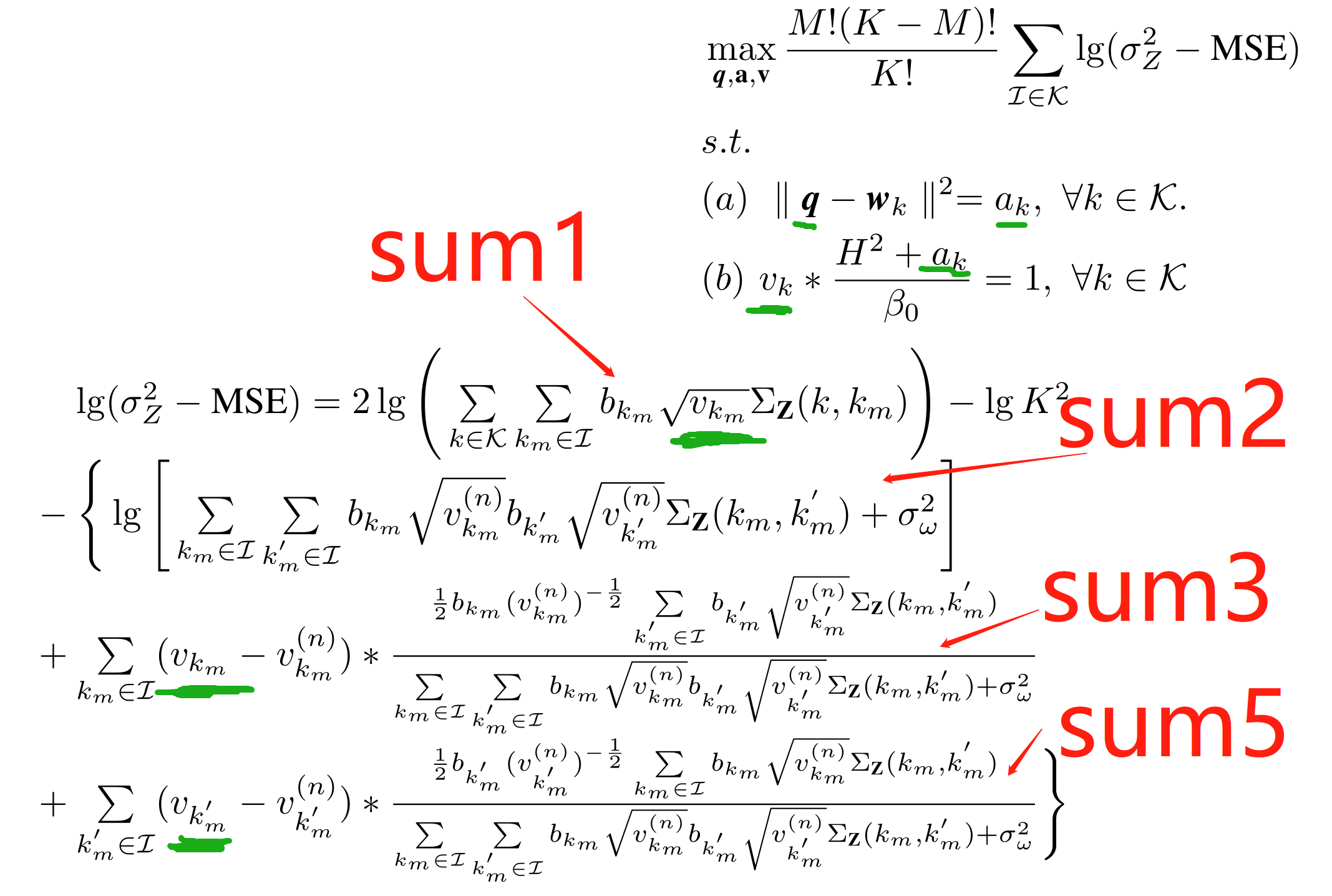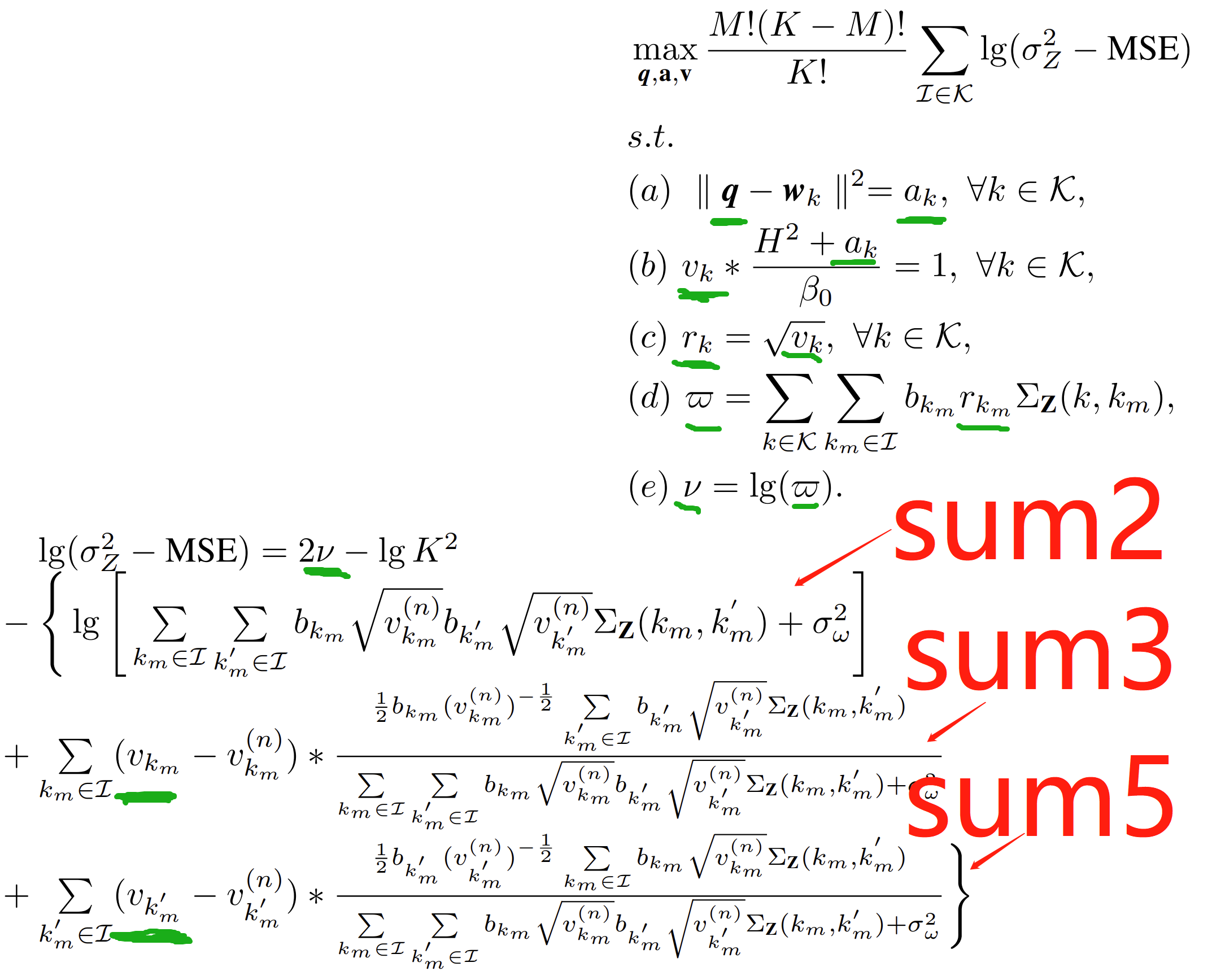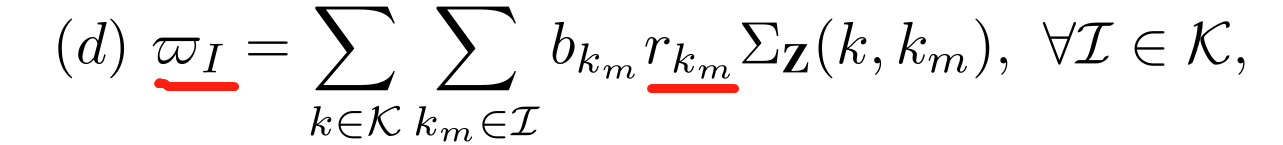•Gurobi Staff

You should edit your post to use a code block and make sure that your code can be copy-pasted and executed resulting in the error you are trying to solve. Please refer to minimal reproducible example for more details.

•from gurobipy import *from numpy import *import numpy as npimport mathimport random#define global variablesglobal KK = 5global MM = 2global ATtheta1ATtheta1=0.42global ATtheta2ATtheta2=2.00global CPtheta1CPtheta1=0.11global CPtheta2CPtheta2=0.68global CStheta1CStheta1=0.05global CStheta2CStheta2=0.62global HH=100global PmaxPmax=10**(-2)  global N0wN0w=10**(-8)*10**(-3)  global beta0beta0=10**(-4)  global thresholdthreshold=10**(-3)#The Positions of K users are given randomlyglobal uposupos = []for i in range(0,K):    upos.append([400*random.random(),400*random.random()])#define distancedef DS(i,j):    return math.sqrt((upos[i]-upos[j])**2+(upos[i]-upos[j])**2)#The maximum value is obtained in order to normalize the distanceglobal DSsetDSset= []global DSmaxfor i in range(0,K):    for j in range(0,K):            DSset.append(DS(i,j))DSmax=max(DSset)  #define functiondef cov(i,j):    return math.exp((-DS(i,j)/DSmax/ATtheta1)**ATtheta2)#initialized variablesb0=math.sqrt(Pmax)*np.mat(ones((K,1)))q0=np.mat([250,250])#create modelmodel = Model("MyModel")#declare variablesa=model.addVars(K,1,lb=0, ub=GRB.INFINITY, vtype=GRB.CONTINUOUS, name="a")q=model.addVars(1,2,lb=0, ub=GRB.INFINITY, vtype=GRB.CONTINUOUS, name="q")#set objective(The objective function is to choose M numbers from 1 to K, and for each combination, calculate the sum7, and finally obtain the sum value of K!/M!/(K-M)! combinations, which is shown as sumvalue. For how to get the combination, we use the depth-first search approach. In bold below are the optimization variables.sumvalue=0  combination=[]def dfs(last_idx, cnt):    if cnt == M:        print(combination)        sum1=0        for k in range(0,K):            for km in range(0,M):                sum1=sum1+b0[combination[km]]*math.sqrt(beta0/(H**2+a[combination[km]]))*cov(k,combination[km])        sum2=0        for km in range(0,M):            for km1 in range(0,M):                sum2=sum2+b0[combination[km]]*math.sqrt(beta0/(H**2+((q0-upos[combination[km]])**2+(q0-upos[combination[km]])**2)))*b0[combination[km1]]*math.sqrt(beta0/(H**2+((q0-upos[combination[km1]])**2+(q0-upos[combination[km1]])**2)))*cov(combination[km],combination[km1])          sum3=0        for km in range(0,M):            sum4=0            for km1 in range(0,M):                sum4=sum4+b0[combination[km1]]*math.sqrt(beta0/(H**2+((q0-upos[combination[km1]])**2+(q0-upos[combination[km1]])**2)))*cov(combination[km],combination[km1])            sum3=sum3+(a[combination[km]]-((q0-upos[combination[km]])**2+(q0-upos[combination[km]])**2))*(-b0[combination[km]]*math.sqrt(beta0))/2/(H**2+(q0-upos[combination[km]])**2+(q0-upos[combination[km]])**2)**(3/2)*sum4/(sum2+N0w)        sum5=0        for km1 in range(0,M):            sum6=0            for km in range(0,M):                sum6=sum6+b0[combination[km]]*math.sqrt(beta0/(H**2+((q0-upos[combination[km]])**2+(q0-upos[combination[km]])**2)))*cov(combination[km],combination[km1])            sum5=sum5+(a[combination[km1]]-((q0-upos[combination[km1]])**2+(q0-upos[combination[km1]])**2))*(-b0[combination[km1]])*math.sqrt(beta0)/2/(H**2+((q0-upos[combination[km1]])**2+(q0-upos[combination[km1]])**2))**(3/2)*sum6/(sum2+N0w)              sum7=2*math.log(sum1)-math.log(K**2)-(math.log(sum2+N0w)+sum3+sum5)        sumvalue=sumvalue+sum7        return    for idx in range(last_idx+1,K):        combination.append(idx)        dfs(idx, cnt+1)        combination.pop()dfs(-1,0)obj=math.factorial(M)*math.factorial(K-M)/math.factorial(K)*sumvaluemodel.setObjective(obj, GRB.MAXIMIZE)model.addConstr(a[k]==(q-upos[k])**2+(q-upos[k])**2 for k in range(0,K))#optimizemodel.optimize()print("Obj: ", model.objVal)opt=model.objVal

•Hi, thank you very much for your comments. For the optimization problem given by the above picture, optimization variables are underlined in green, and we use Gurobi to sove the problem. According to your comments, we have given the complete code, looking forward to your reply, many thanks!

All the best,

Yali

•Gurobi Staff

Hi Yali,

$$\texttt{a}$$ is a tupledict of Var objects returned by the addVars method and cannot be accessed via two indices but rather by a tuple, i.e., you have to access the entries of $$\texttt{a}$$ via $$\texttt{a[i,j]}$$ instead of $$\texttt{a[i][j]}$$. The same applies to your variables $$\texttt{q}$$.

You will also run into the error

  File "test3.py", line 72, in dfs    sum1=sum1+b0[combination[km]]*math.sqrt(beta0/(H**2+a[combination[km],0]))*cov(k,combination[km])  File "src/gurobipy/linexpr.pxi", line 570, in gurobipy.LinExpr.__truediv__gurobipy.GurobiError: Divisor must be a constant

Gurobi currently does not support division by a variable. However, we discuss a workaround in the Knowledge Base article How do I divide by a variable in Gurobi?

Best regards,
Jaromił

•Hi Jaromił, thanks for your help!

All the best,

Yali

•Hi Jaromił, according to your comments, we have changed the code, but there are still some problems. Looking forward to your reply! Many thanks!

We use Gurobi to sove the following problem. Optimization variables are underlined in green.Then, I will attach my complete code below. sum1=sum1+b0[combination[km]]*math.sqrt(v[combination[km]])*cov(k,combination[km])
TypeError: must be real number, not Var

from gurobipy import *from numpy import *import numpy as npimport mathimport random#define global variablesglobal KK = 5global MM = 2global ATtheta1ATtheta1=0.42global ATtheta2ATtheta2=2.00global CPtheta1CPtheta1=0.11global CPtheta2CPtheta2=0.68global CStheta1CStheta1=0.05global CStheta2CStheta2=0.62global HH=100global PmaxPmax=10**(-2)  global N0wN0w=10**(-8)*10**(-3)  global beta0beta0=10**(-4)  global thresholdthreshold=10**(-3)#The Positions of K users are given randomlyglobal uposupos = []for i in range(0,K):    upos.append([400*random.random(),400*random.random()])#define distancedef DS(i,j):    return math.sqrt((upos[i]-upos[j])**2+(upos[i]-upos[j])**2)#The maximum value is obtained in order to normalize the distanceglobal DSsetDSset= []global DSmaxfor i in range(0,K):    for j in range(0,K):            DSset.append(DS(i,j))DSmax=max(DSset)  #define functiondef cov(i,j):    return math.exp((-DS(i,j)/DSmax/ATtheta1)**ATtheta2)#initialized variablesb0=math.sqrt(Pmax)*np.mat(ones((K)))q0=np.mat([250,250])#create modelmodel = Model("MyModel")#declare variablesq=model.addVars(2, lb=0, ub=400, vtype=GRB.CONTINUOUS, name="q")a=model.addVars(K, name="a")v=model.addVars(K, name="v")sumvariable=model.addVars(1, name="sumvariable")#set objective(The objective function is to choose M numbers from 1 to K, and for each combination, calculate the sum7, and finally obtain the sum value of K!/M!/(K-M)! combinations, which is shown as sumvalue. For how to get the combination, we use the depth-first search approach. In bold below are the optimization variables.sumvalue=0  combination=[]def dfs(last_idx, cnt):    if cnt == M:        print(combination)        sum1=0        for k in range(0,K):            for km in range(0,M):                sum1=sum1+b0[combination[km]]*math.sqrt(v[combination[km]])*cov(k,combination[km])        #Since lg(sum1) is a component of the objective function, we assign sum1 to a defined variable, that is sumvariable        sumvariable=sum1                sum2=0        for km in range(0,M):            for km1 in range(0,M):                sum2=sum2+b0[combination[km]]*math.sqrt(beta0/(H**2+(q0-upos[combination[km]])**2+(q0-upos[combination[km]])**2))*b0[combination[km1]]*math.sqrt(beta0/(H**2+(q0-upos[combination[km1]])**2+(q0-upos[combination[km1]])**2))*cov(combination[km],combination[km1])          sum3=0        for km in range(0,M):            sum4=0            for km1 in range(0,M):                sum4=sum4+b0[combination[km1]]*math.sqrt(beta0/(H**2+(q0-upos[combination[km1]])**2+(q0-upos[combination[km1]])**2))*cov(combination[km],combination[km1])            sum3=sum3+(v[combination[km]]-(beta0/(H**2+(q0-upos[combination[km]])**2+(q0-upos[combination[km]])**2)))*1/2*b0[combination[km]]*(beta0/(H**2+(q0-upos[combination[km]])**2+(q0-upos[combination[km]])**2))**(-1/2)*sum4/(sum2+N0w)                sum5=0        for km1 in range(0,M):            sum6=0            for km in range(0,M):                sum6=sum6+b0[combination[km]]*math.sqrt(beta0/(H**2+(q0-upos[combination[km]])**2+(q0-upos[combination[km]])**2))*cov(combination[km],combination[km1])            sum5=sum5+(v[combination[km1]]-(beta0/(H**2+(q0-upos[combination[km1]])**2+(q0-upos[combination[km1]])**2)))*1/2*b0[combination[km1]]*(beta0/(H**2+(q0-upos[combination[km1]])**2+(q0-upos[combination[km1]])**2))**(-1/2)*sum6/(sum2+N0w)              sum7=2*math.log(sumvariable)-math.log(K**2)-(math.log(sum2+N0w)+sum3+sum5)        sumvalue=sumvalue+sum7        return    for idx in range(last_idx+1,K):        combination.append(idx)        dfs(idx, cnt+1)        combination.pop()dfs(-1,0)obj=math.factorial(M)*math.factorial(K-M)/math.factorial(K)*sumvaluemodel.setObjective(obj, GRB.MAXIMIZE)model.addConstr(a[k]==(q-upos[k])**2+(q-upos[k])**2 for k in range(0,K))model.addConstr(v[k]*(H**2+a[k])/beta0 == 1 for k in range(0,K))model.params.NonConvex=2#optimizemodel.optimize()print("Obj: ", model.objVal)opt=model.objVal
•Gurobi Staff

Hi Yali,

you are calling the $$\sqrt{}$$ function from the $$\texttt{math}$$ package on a Gurobi Var. This is not possible. You have to use Gurobi's general constraints feature to implement nonlinear functions which have optimization variables or expressions holding optimization variables as input. Note that Gurobi currently uses a piecewise-linear approximation of the nonlinear functions such that the final solution will very likely suffer from some (small) approximation error.

In order to model the particular function $$\sqrt{f(x)}$$ where $$x$$ is an optimization variable, you have to introduce an auxiliary variable for the argument variable and for the result. This means that you have to model

\begin{align*} w_1 & = f(x)\\ w_2 & = \sqrt{w_1} \end{align*}

with appropriate bounds for the auxiliary variables $$w_1, w_2$$. You can then use $$w_2$$ in the rest of your model. In the particular case of the $$\sqrt{}$$ function, you can use the addGenConstrPow method with $$a=0.5$$. You can find the list of all supported general functions here.

Best regards,
Jaromił

•Hi Jaromił, according to your comments, we have changed the code, but there are still some problems. TypeError: unsupported operand type(s) for *: 'int' and 'tupledict'：sum7=2*nu-math.log(K**2)-(math.log(sum2+N0w)+sum3+sum5). Looking forward to your reply! Many thanks!

We use Gurobi to sove the following problem. Optimization variables are underlined in green.from gurobipy import *from numpy import *import numpy as npimport mathimport random#define global variablesglobal KK = 5global MM = 2global ATtheta1ATtheta1=0.42global ATtheta2ATtheta2=2.00global CPtheta1CPtheta1=0.11global CPtheta2CPtheta2=0.68global CStheta1CStheta1=0.05global CStheta2CStheta2=0.62global HH=100global PmaxPmax=10**(-2)  global N0wN0w=10**(-8)*10**(-3)  global beta0beta0=10**(-4)  global thresholdthreshold=10**(-3)#The Positions of K users are given randomlyglobal uposupos = []for i in range(0,K):    upos.append([400*random.random(),400*random.random()])#define distancedef DS(i,j):    return math.sqrt((upos[i]-upos[j])**2+(upos[i]-upos[j])**2)#The maximum value is obtained in order to normalize the distanceglobal DSsetDSset= []global DSmaxfor i in range(0,K):    for j in range(0,K):            DSset.append(DS(i,j))DSmax=max(DSset)  #define functiondef cov(i,j):    return math.exp((-DS(i,j)/DSmax/ATtheta1)**ATtheta2)#initialized variablesb0=math.sqrt(Pmax)*np.array(ones((K)))q0=np.array([250,250])#create modelmodel = Model("MyModel")#declare variablesq=model.addVars(2, lb=0, ub=400, vtype=GRB.CONTINUOUS, name="q")a=model.addVars(K, name="a")v=model.addVars(K, name="v")r=model.addVars(K, name="r")varpi=model.addVars(1, name="varpi")nu=model.addVars(1, name="nu")#set objective(The objective function is to choose M numbers from 1 to K, and for each combination, calculate the sum7, and finally obtain the sum value of K!/M!/(K-M)! combinations, which is shown as sumvalue. For how to get the combination, we use the depth-first search approach. In bold below are the optimization variables.sumvalue=0  combination=[]def dfs(last_idx, cnt):    if cnt == M:        print(combination)            sum2=0        for km in range(0,M):            for km1 in range(0,M):                sum2=sum2+b0[combination[km]]*math.sqrt(beta0/(H**2+(q0-upos[combination[km]])**2+(q0-upos[combination[km]])**2))*b0[combination[km1]]*math.sqrt(beta0/(H**2+(q0-upos[combination[km1]])**2+(q0-upos[combination[km1]])**2))*cov(combination[km],combination[km1])          sum3=0        for km in range(0,M):            sum4=0            for km1 in range(0,M):                sum4=sum4+b0[combination[km1]]*math.sqrt(beta0/(H**2+(q0-upos[combination[km1]])**2+(q0-upos[combination[km1]])**2))*cov(combination[km],combination[km1])            sum3=sum3+(v[combination[km]]-(beta0/(H**2+(q0-upos[combination[km]])**2+(q0-upos[combination[km]])**2)))*1/2*b0[combination[km]]*(beta0/(H**2+(q0-upos[combination[km]])**2+(q0-upos[combination[km]])**2))**(-1/2)*sum4/(sum2+N0w)                sum5=0        for km1 in range(0,M):            sum6=0            for km in range(0,M):                sum6=sum6+b0[combination[km]]*math.sqrt(beta0/(H**2+(q0-upos[combination[km]])**2+(q0-upos[combination[km]])**2))*cov(combination[km],combination[km1])            sum5=sum5+(v[combination[km1]]-(beta0/(H**2+(q0-upos[combination[km1]])**2+(q0-upos[combination[km1]])**2)))*1/2*b0[combination[km1]]*(beta0/(H**2+(q0-upos[combination[km1]])**2+(q0-upos[combination[km1]])**2))**(-1/2)*sum6/(sum2+N0w)              sum7=2*nu-math.log(K**2)-(math.log(sum2+N0w)+sum3+sum5)        sumvalue=sumvalue+sum7        return    for idx in range(last_idx+1,K):        combination.append(idx)        dfs(idx, cnt+1)        combination.pop()dfs(-1,0)obj=math.factorial(M)*math.factorial(K-M)/math.factorial(K)*sumvaluemodel.setObjective(obj, GRB.MAXIMIZE)model.addConstr(a[k]==(q-upos[k])**2+(q-upos[k])**2 for k in range(0,K))model.addConstr(v[k]*(H**2+a[k])/beta0 == 1 for k in range(0,K))for k in range(0,K):    model.addGenConstrPow(v[k], r[k], 0.5)varpi=LinExpr(0)for k in range(0,K):    for km in range(0,M):        varpi.addTerms(1, b0[combination[km]]*r[combination[km]]*cov(k,combination[km]))model.addGenConstrLogA(varpi, nu, 10.0)model.params.NonConvex=2#optimizemodel.optimize()print("Obj: ", model.objVal)opt=model.objVal
•Gurobi Staff

The errror is caused because the $$\texttt{nu}$$ is a tupledict of Var objects returned by the addVars method and you have to access its indices. In this case, this would be $$\texttt{nu}$$. It holds only 1 variable so it is probably better to just use the addVar method, then you don't have to change the code for $$\texttt{sum7}$$. The same holds for the optimization variable $$\texttt{varpi}$$.

•Hi Jaromił, sorry to bother you again. IndexError: list index out of range: constr_1.addTerms(1, b0[combination[km]]*r[combination[km]]*cov(k,combination[km])).

from gurobipy import *from numpy import *import numpy as npimport mathimport random#define global variablesglobal KK = 5global MM = 2global ATtheta1ATtheta1=0.42global ATtheta2ATtheta2=2.00global CPtheta1CPtheta1=0.11global CPtheta2CPtheta2=0.68global CStheta1CStheta1=0.05global CStheta2CStheta2=0.62global HH=100global PmaxPmax=10**(-2)  global N0wN0w=10**(-8)*10**(-3)  global beta0beta0=10**(-4)  global thresholdthreshold=10**(-3)#The Positions of K users are given randomlyglobal uposupos = []for i in range(0,K):    upos.append([400*random.random(),400*random.random()])#define distancedef DS(i,j):    return math.sqrt((upos[i]-upos[j])**2+(upos[i]-upos[j])**2)#The maximum value is obtained in order to normalize the distanceglobal DSsetDSset= []global DSmaxfor i in range(0,K):    for j in range(0,K):            DSset.append(DS(i,j))DSmax=max(DSset)  #define functiondef cov(i,j):    return math.exp((-DS(i,j)/DSmax/ATtheta1)**ATtheta2)#initialized variablesb0=math.sqrt(Pmax)*np.array(ones((K)))q0=np.array([250,250])#create modelmodel = Model("MyModel")#declare variablesq=model.addVars(2, lb=0, ub=400, vtype=GRB.CONTINUOUS, name="q")a=model.addVars(K, name="a")v=model.addVars(K, name="v")r=model.addVars(K, name="r")varpi=model.addVars(1, name="varpi")nu=model.addVars(1, name="nu")#set objective(The objective function is to choose M numbers from 1 to K, and for each combination, calculate the sum7, and finally obtain the sum value of K!/M!/(K-M)! combinations, which is shown as sumvalue. For how to get the combination, we use the depth-first search approach. In bold below are the optimization variables.sumvalue=0  combination=[]def dfs(last_idx, cnt):    global sumvalue    if cnt == M:        print(combination)            sum2=0        for km in range(0,M):            for km1 in range(0,M):                sum2=sum2+b0[combination[km]]*math.sqrt(beta0/(H**2+(q0-upos[combination[km]])**2+(q0-upos[combination[km]])**2))*b0[combination[km1]]*math.sqrt(beta0/(H**2+(q0-upos[combination[km1]])**2+(q0-upos[combination[km1]])**2))*cov(combination[km],combination[km1])          sum3=0        for km in range(0,M):            sum4=0            for km1 in range(0,M):                sum4=sum4+b0[combination[km1]]*math.sqrt(beta0/(H**2+(q0-upos[combination[km1]])**2+(q0-upos[combination[km1]])**2))*cov(combination[km],combination[km1])            sum3=sum3+(v[combination[km]]-(beta0/(H**2+(q0-upos[combination[km]])**2+(q0-upos[combination[km]])**2)))*1/2*b0[combination[km]]*(beta0/(H**2+(q0-upos[combination[km]])**2+(q0-upos[combination[km]])**2))**(-1/2)*sum4/(sum2+N0w)                sum5=0        for km1 in range(0,M):            sum6=0            for km in range(0,M):                sum6=sum6+b0[combination[km]]*math.sqrt(beta0/(H**2+(q0-upos[combination[km]])**2+(q0-upos[combination[km]])**2))*cov(combination[km],combination[km1])            sum5=sum5+(v[combination[km1]]-(beta0/(H**2+(q0-upos[combination[km1]])**2+(q0-upos[combination[km1]])**2)))*1/2*b0[combination[km1]]*(beta0/(H**2+(q0-upos[combination[km1]])**2+(q0-upos[combination[km1]])**2))**(-1/2)*sum6/(sum2+N0w)              sum7=2*nu-math.log(K**2)-(math.log(sum2+N0w)+sum3+sum5)        sumvalue=sumvalue+sum7        return    for idx in range(last_idx+1,K):        combination.append(idx)        dfs(idx, cnt+1)        combination.pop()dfs(-1,0)obj=math.factorial(M)*math.factorial(K-M)/math.factorial(K)*sumvaluemodel.setObjective(obj, GRB.MAXIMIZE)model.addConstrs(a[k]==(q-upos[k])**2+(q-upos[k])**2 for k in range(0,K))model.addConstrs(v[k]*(H**2+a[k])/beta0 == 1 for k in range(0,K))for k in range(0,K):    model.addGenConstrPow(v[k], r[k], 0.5)constr_1 = LinExpr(0)for k in range(0,K):    for km in range(0,M):        constr_1.addTerms(1, b0[combination[km]]*r[combination[km]]*cov(k,combination[km]))  model.addConstr(varpi == constr_1)model.addGenConstrLogA(varpi, nu, 10.0)model.params.NonConvex=2#optimizemodel.optimize()print("Obj: ", model.objVal)opt=model.objVal

All the best,

Yali

•Gurobi Staff

At that line of code, $$\texttt{combination}$$ is an empty list, so you cannot access the list at index $$\texttt{km}$$ (here, $$\texttt{km}$$ is $$\texttt{0}$$). If you're expecting the $$\texttt{combination}$$ list to be nonempty at this point, there must be an issue in how you construct $$\texttt{combination}$$.

Your $$\texttt{dfs}$$ function:

def dfs(last_idx, cnt):    ...    for idx in range(last_idx+1,K):        combination.append(idx)        dfs(idx, cnt+1)        combination.pop()

Here, elements are being added to $$\texttt{combination}$$, the function is called recursively, and then the last element is removed from $$\texttt{combination}$$.

•Hi Eli, sorry to bother you again.  According to your comments about the code I showed above，the error is in the following part，

constr_1 = LinExpr(0)for k in range(0,K):    for km in range(0,M):        constr_1.addTerms(1, b0[combination[km]]*r[combination[km]]*cov(k,combination[km]))  model.addConstr(varpi == constr_1)
First, let me introduce you to the details. We choose M numbers from 1 to K, and for each combination, calculate the sum7, and finally obtain the sum value of K!/M!/(K-M)! combinations, which is shown as sumvalue. For how to get the combination, we use the depth-first search approach. Now，if the constraint corresponding to the error code is changed as follows:Optimization variables are underlined in red. For each combination, we need to write this constraint, so please tell me how should I add it to the my dfs function? Where it should be added is before the optimization objective

model.setObjective(obj, GRB.MAXIMIZE)

appears, but it is a constraint so I don't understand how to add it.
In addition, you also know that M numbers are selected from 1 to K. If K=50, the number of combinations will be very large, and the computational complexity of this code will be very high, which is why we use depth-first search. But in this constraint, for each combination, the equation must be satisfied, which also involves complexity issues, we cannot directly list the constraints for each combination. I really want to ask you these questions, looking forward to your reply！Many thanks！

All the best，

Yali

•Gurobi Staff

I see you created a follow-up post The nonlinear function in the optimization objective contains optimization variables with this same question. We can continue the discussion there.